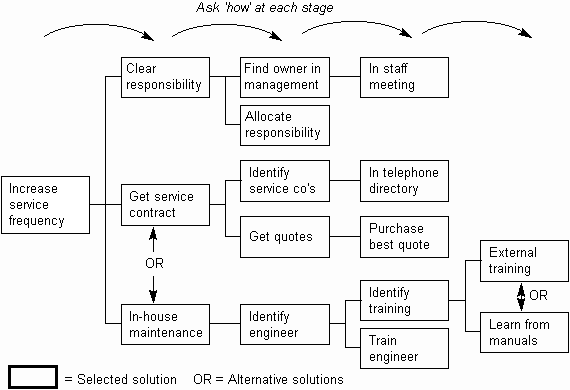The Psychology of Quality and More

# How-How Diagram

A How-How Diagram is a Tree Diagram where each child is determined by asking 'how' the parent can be achieved, as below. It is thus useful for creating solutions to problems.

The Why-Why and How-How Diagrams together make a very simple toolset for finding causes of and solutions to problems.Why-why Diagram, Tree Diagram

### You can buy books here

And the big
paperback book